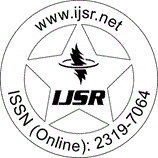International Journal of Science and Research (IJSR)
Call for Papers | Fully Refereed | Open Access | Double Blind Peer Reviewed

Research Paper | Mathematics | India | Volume 5 Issue 1, January 2016

# Solving Hybrid Fuzzy Fractional Differential Equations by Milne's Method

S. Ruban Raj  | M. Saradha 

Abstract: In this paper we study numerical methods for hybrid fuzzy fractional differential equations and the iteration method is used to solve the hybrid fuzzy fractional differential equations with a fuzzy initial condition. We consider a differential equation of fractional order and we compared the results with their exact solutions in order to demonstrate the validity and applicability of the method. We further give the definition of the Degree of Sub element hood of hybrid fuzzy fractional differential equations with examples.

Keywords: hybrid fuzzy fractional differential equations, degree of sub element hood

Edition: Volume 5 Issue 1, January 2016,

Pages: 47 - 50